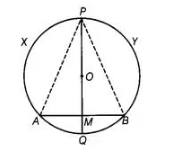# If the perpendicular bisector of a chord`
Question:

If the perpendicular bisector of a chord $A B$ of a circle $P X A Q B Y$ intersects the circle at $P$ and $Q$, prove that arc $P X A=\operatorname{arc} P Y B$.

Thinking Process

Firstly, prove that $\triangle \mathrm{APM}$ is congruent to $\triangle \mathrm{BPM}$ by SAS rule, then further prove the required result by CPCT rule.

Solution:

Let $A B$ be a chord of a circle having centre at $O P Q$ be the perpendicular bisector of the chord $A B$, which intersects at $M$ and it always passes through $O$.To prove arc PXA ≅ arc PYB

Construction Join AP and BP.

Proof In ΔAPM and ΔBPM,

AM = MB

∠PMA = ∠PMB

PM = PM

∴ ΔAPM s ΔBPM

∴PA = PB

=> arc PXA ≅ arc PYB